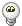Jump to content
• Sign Up

## Recommended Posts

I have a program which logs in unix time. When I try to convert unix time to normal in autoit, it gives me time with +4 hours. I've tried using _DateAdd() from Date.au3 UDF and I've tried trancexx's _EPOCH_decrypt()from here: Link

```\$unixtime = 1272700408

; Example 1
#include <date.au3>
\$iDateCalc = _DateAdd('s', \$unixtime, "1970/01/01 00:00:00")
ConsoleWrite('\$iDateCalc = ' & \$iDateCalc & @crlf)

;Exaple 2
ConsoleWrite("EPOCH time 1272700408 is " & _Epoch_decrypt(1272700408) & @CRLF)
Func _EPOCH_decrypt(\$epoch_time)
Local \$Day2Add = Int(\$epoch_time / 86400)
Local \$iTimeVal = Mod(\$epoch_time, 86400)
If \$iTimeVal < 0 Then
\$Day2Add -= 1
\$iTimeVal += 86400
EndIf
Local \$i_wFactor = Int((573371.75 + \$Day2Add) / 36524.25)
Local \$i_xFactor = Int(\$i_wFactor / 4)
Local \$i_bFactor = 2442113 + \$Day2Add + \$i_wFactor - \$i_xFactor
Local \$i_cFactor = Int((\$i_bFactor - 122.1) / 365.25)
Local \$i_dFactor = Int(365.25 * \$i_cFactor)
Local \$i_eFactor = Int((\$i_bFactor - \$i_dFactor) / 30.6001)
Local \$asDatePart
\$asDatePart = \$i_bFactor - \$i_dFactor - Int(30.6001 * \$i_eFactor)
\$asDatePart = \$i_eFactor - 1 - 12 * (\$i_eFactor - 2 >= 13)
\$asDatePart = \$i_cFactor - 4716 + (\$asDatePart < 3)
Local \$asTimePart
\$asTimePart = Int(\$iTimeVal / 3600)
\$iTimeVal = Mod(\$iTimeVal, 3600)
\$asTimePart = Int(\$iTimeVal / 60)
\$asTimePart = Mod(\$iTimeVal, 60)
Return SetError(0, 0, StringFormat("%.2d/%.2d/%.2d %.2d:%.2d:%.2d", \$asDatePart, \$asDatePart, \$asDatePart, \$asTimePart, \$asTimePart, \$asTimePart))
EndFunc```

The results are:

```\$iDateCalc = 2010/05/01 07:53:28
EPOCH time 1272700408 is 2010/05/01 07:53:28```

They should be: 2010/05/01 03:53:28

The program I'm talking about is SABnzbd. It converts the unix time to normal time correctly. Now the question is, which one is at fault? Is autoit wrong in calculating, or does SABnzbd provide a wrong unix time to begin with?

Edited by shEiD

#### Share this post

##### Share on other sites

Maybe you are not taking your timezone into account? For example for Newyork:

UTC/GMT Offset

Standard time zone: UTC/GMT -5 hours

Daylight saving time: +1 hour

Current time zone offset: UTC/GMT -4 hours

Time zone abbreviation: EDT - Eastern Daylight Time

Jos

SciTE4AutoIt3 Full installer Download page   - Beta files

Live for the present,
Dream of the future,
Learn from the past.#### Share this post

##### Share on other sites

Maybe you are not taking your timezone into account? For example for Newyork:

UTC/GMT Offset

Standard time zone: UTC/GMT -5 hours

Daylight saving time: +1 hour

Current time zone offset: UTC/GMT -4 hours

Time zone abbreviation: EDT - Eastern Daylight Time

Jos

Thank you. So dumb of meI'm exactly in New YorkWould this be the proper/correct way of doing this:

```#include <date.au3>
\$unixtime = 1272700408
\$timezone = _Date_Time_GetTimeZoneInformation()
\$unixtime = \$unixtime - (\$timezone + \$timezone) * 60
\$iDateCalc = _DateAdd('s', \$unixtime, "1970/01/01 00:00:00")```

This gives me the correct time. I'm just asking, will this work correctly in any timezone and on any daylight savings settings? I'm sorry, I have no experience in this thing, that's the first time I've run into timezone/daylight situation#### Share this post

##### Share on other sites

_DateAdd() VS _EPOCH_decrypt() test shows that _EPOCH_decrypt() is 6-7 times faster.

```#include <Timers.au3>
#include <date.au3>
Global \$timer
Global \$times = 100000
Global \$vs1 = "_DateAdd()"
Global \$vs2 = "_EPOCH_decrypt()"
\$unixtime = 1272700408
\$timezone = _Date_Time_GetTimeZoneInformation()
\$unixtime = \$unixtime - (\$timezone + \$timezone) * 60
; First
\$timer = _Timer_Init()
For \$i = 1 To \$times
\$date = _DateAdd('s', \$unixtime, "1970/01/01 00:00:00")
Next
ConsoleWrite(_TimeStamp(_Timer_Diff(\$timer)) & \$vs1 & @CRLF)
; Second
\$timer = _Timer_Init()
For \$i = 1 To \$times
\$date = _Epoch_decrypt(\$unixtime)
Next
ConsoleWrite(_TimeStamp(_Timer_Diff(\$timer)) & \$vs2 & @CRLF)
Func _EPOCH_decrypt(\$epoch_time)
Local \$Day2Add = Int(\$epoch_time / 86400)
Local \$iTimeVal = Mod(\$epoch_time, 86400)
If \$iTimeVal < 0 Then
\$Day2Add -= 1
\$iTimeVal += 86400
EndIf
Local \$i_wFactor = Int((573371.75 + \$Day2Add) / 36524.25)
Local \$i_xFactor = Int(\$i_wFactor / 4)
Local \$i_bFactor = 2442113 + \$Day2Add + \$i_wFactor - \$i_xFactor
Local \$i_cFactor = Int((\$i_bFactor - 122.1) / 365.25)
Local \$i_dFactor = Int(365.25 * \$i_cFactor)
Local \$i_eFactor = Int((\$i_bFactor - \$i_dFactor) / 30.6001)
Local \$asDatePart
\$asDatePart = \$i_bFactor - \$i_dFactor - Int(30.6001 * \$i_eFactor)
\$asDatePart = \$i_eFactor - 1 - 12 * (\$i_eFactor - 2 >= 13)
\$asDatePart = \$i_cFactor - 4716 + (\$asDatePart < 3)
Local \$asTimePart
\$asTimePart = Int(\$iTimeVal / 3600)
\$iTimeVal = Mod(\$iTimeVal, 3600)
\$asTimePart = Int(\$iTimeVal / 60)
\$asTimePart = Mod(\$iTimeVal, 60)
Return SetError(0, 0, StringFormat("%.2d/%.2d/%.2d %.2d:%.2d:%.2d", \$asDatePart, \$asDatePart, \$asDatePart, \$asTimePart, \$asTimePart, \$asTimePart))
EndFunc   ;==>_EPOCH_decrypt
Func _TimeStamp(\$tdif)
Local \$msg = ";"
\$msg &= StringFormat("%10s", "*" & \$times)
\$msg &= StringFormat("%10s", Round(\$tdif) & "ms")
\$msg &= StringFormat("%7s", Round(\$tdif / 1000) & "s") & "  -> "
Return \$msg
EndFunc   ;==>_TimeStamp```

The results:

```;      *100      39ms     0s  -> _DateAdd()
;      *100       6ms     0s  -> _EPOCH_decrypt()
;     *1000     345ms     0s  -> _DateAdd()
;     *1000      50ms     0s  -> _EPOCH_decrypt()
;    *10000    3290ms     3s  -> _DateAdd()
;    *10000     515ms     1s  -> _EPOCH_decrypt()
;   *100000   33410ms    33s  -> _DateAdd()
;   *100000    5174ms     5s  -> _EPOCH_decrypt()```

## Create an account or sign in to comment

You need to be a member in order to leave a comment

## Create an account

Sign up for a new account in our community. It's easy!

Register a new account

## Sign in

Already have an account? Sign in here.

Sign In Now

• ### Recently Browsing   0 members

No registered users viewing this page.

×

• Wiki

• Back

• #### Beta

• Git
• FAQ
• Our Picks
×
• Create New...